Question

# (5) a) Sketch r = 3+ 3 cosθ and b) Find the are length of the curve for 2π/3 ≤ θ ≤ π

(5) a) Sketch r = 3+ 3 cosθ and b) Find the are length of the curve for 2π/3 ≤ θ ≤ π

As you've asked to solve the part (b), therefore, I'm solving that part.

Solution: The arc length of a polar curve r = f(θ) between θ = a and θ = b is given by the integral: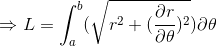(1)

Given the curve: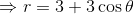(2)

Differentiating this equation w.r.t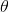, we'll get: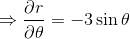(3)

Since we've to calculate the length of the polar between,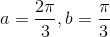(4)

in equation (1). Thus, we've to calculate,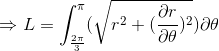Using (1), (2), (3) and (4), we'll get: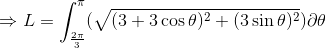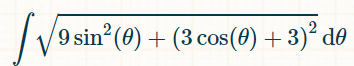On simplifying, we'll get: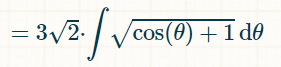Now solving,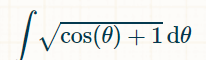Rewriting using trigonometric identity,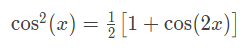We'll get: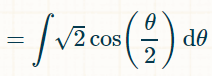On integration, we'll get: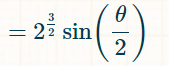Therefore,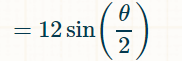Substituting limits, we'll get: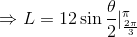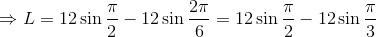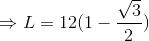I hope it helps you!

#### Earn Coins

Coins can be redeemed for fabulous gifts.

Similar Homework Help Questions
• ### Find the exact length of the polar curve. r=θ₂, 0≤θ≤π/2

Find the exact length of the polar curve. r=θ₂, 0≤θ≤π/2

• ### Stir or the curve r θ; from θ 0 to θ 2π. 8. Find the eccentricity and classify the conic, and sketch the graph labeling the vertices. 12 6-2sin(8) Find the eccentricity and classify the conic, an...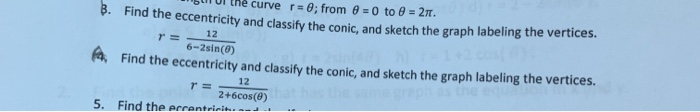Stir or the curve r θ; from θ 0 to θ 2π. 8. Find the eccentricity and classify the conic, and sketch the graph labeling the vertices. 12 6-2sin(8) Find the eccentricity and classify the conic, and sketch the graph labeling the vertices. Find the errentricit 5. Stir or the curve r θ; from θ 0 to θ 2π. 8. Find the eccentricity and classify the conic, and sketch the graph labeling the vertices. 12 6-2sin(8) Find the eccentricity and...

• ### Sketch the curve: r = 1- cosθ .

Sketch the curve: r = 1- cosθ . Sketch the curv:e r = -2 sinθ. Sketch the curve: r = 3 cos 3θ.

• ### A curve in polar coordinates is given by: r = 9 + 2 cos θ Point P is at θ = 20π/18

A curve in polar coordinates is given by: r = 9 + 2 cos θ Point P is at θ = 20π/18 (1) Find polar coordinate r for P, with r > 0 and π < θ < 3π/2. (2) Find cartesian coordinates for point P (3) How may times does the curve pass through the origin when 0 < θ < 2π?

• ### (10 marks) In class we had a question regarding the spherical coordinate system: Given that rcos θ sin φ y-rsin0 sin o with 0 θ 2π and 0 φ π "Why don't we have 0 θ π and 0 φ 2π instead&#3...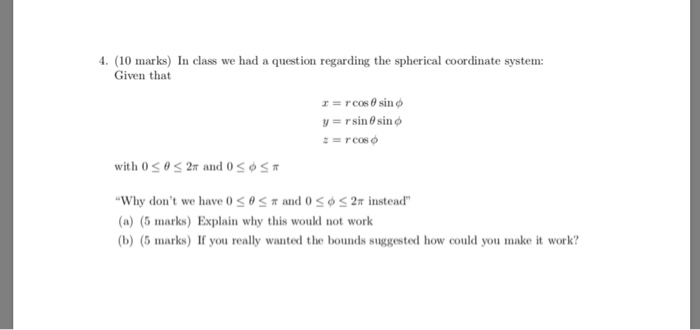(10 marks) In class we had a question regarding the spherical coordinate system: Given that rcos θ sin φ y-rsin0 sin o with 0 θ 2π and 0 φ π "Why don't we have 0 θ π and 0 φ 2π instead" (a) (5 marks) Explain why this would not work b) (5 marks) If you really wanted the bounds suggested how could you make it work? (10 marks) In class we had a question regarding the spherical coordinate system:...

• ### 5. (a) (6) Carefully sketch the odd periodic extension, of period 2m, of the function f(x)1, 0 < x < π. (Only sketch over the interval z E [-2π, 2π). (b) (10) Find the Fourier sine series o...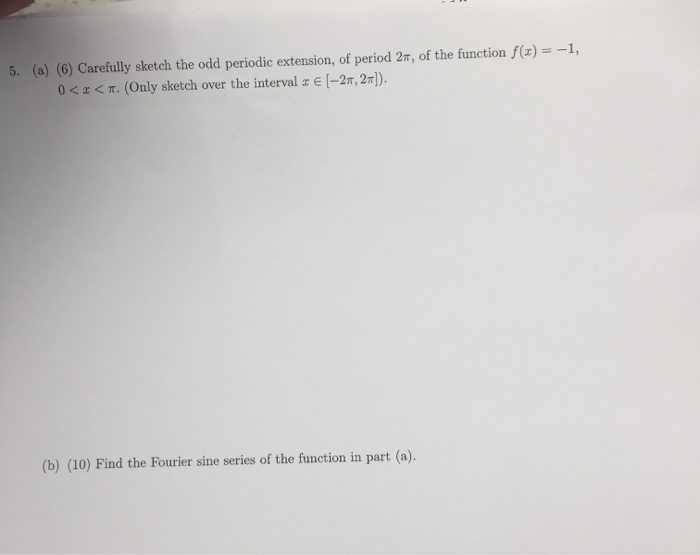5. (a) (6) Carefully sketch the odd periodic extension, of period 2m, of the function f(x)1, 0 < x < π. (Only sketch over the interval z E [-2π, 2π). (b) (10) Find the Fourier sine series of the function in part (a) 5. (a) (6) Carefully sketch the odd periodic extension, of period 2m, of the function f(x)1, 0

• ### Suppose f is integrable on (-π, π] and extended to R by making it periodic of period 2π. Show that f(x) dx= | f(x)dz where I is any interval in R of length 2π Hint: I is contained in two consecutive...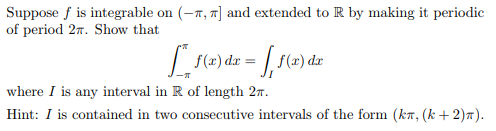Suppose f is integrable on (-π, π] and extended to R by making it periodic of period 2π. Show that f(x) dx= | f(x)dz where I is any interval in R of length 2π Hint: I is contained in two consecutive intervals of the form (kT, (k+2)π) Suppose f is integrable on (-π, π] and extended to R by making it periodic of period 2π. Show that f(x) dx= | f(x)dz where I is any interval in R of length...

• ### 5. Solve Au=0, r>1, 0 < θ < 2π, a(1,0) cos θ, 0 < θ < 2π. 5. Solve Au=0, r>1, 0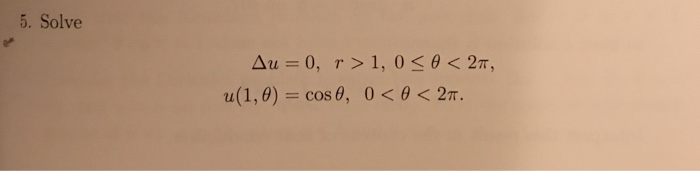5. Solve Au=0, r>1, 0 < θ < 2π, a(1,0) cos θ, 0 < θ < 2π. 5. Solve Au=0, r>1, 0

• ### 5. Solve Au=0, r>1, 0 < θ < 2π, u(1.0) = cos θ, 0 < θ < 2π. 5. Solve Au=0, r>1, 0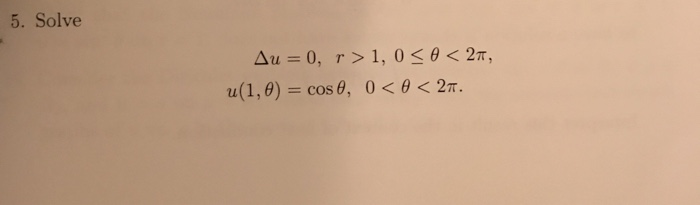5. Solve Au=0, r>1, 0 < θ < 2π, u(1.0) = cos θ, 0 < θ < 2π. 5. Solve Au=0, r>1, 0

• ### all parts please PART II 7) (8 pts) Given the polar equation r = 6 sin θ, 0 θ π a) Graph and find the length of the graph geometrically. b) Find the length of the graph by integrating. 8.) (9 p...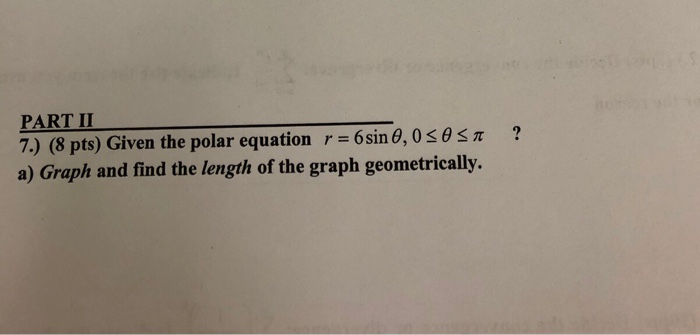all parts please PART II 7) (8 pts) Given the polar equation r = 6 sin θ, 0 θ π a) Graph and find the length of the graph geometrically. b) Find the length of the graph by integrating. 8.) (9 pts) Given the four-leaved rose r 2sin(26). a) Show the symmetries. b) Find the tangents of the leaf through the pole to determine the limits of integration. c) Find the area of one leaf. PART II 7) (8 pts)...